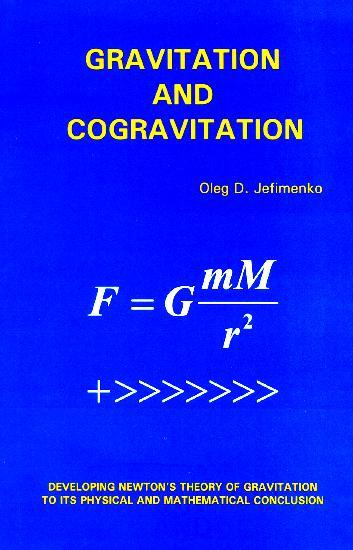# Gravitation and Cogravitation

### Paperback - List Price US\$ 22.00 ISBN 0-917406-15-x

Paperback is sold out. Please order the hardback.

### Hardback - List Price US\$ 32.00 ISBN 0-917406-00-1Newton's theory of gravitation is the grandest and the most enduring physical theory ever created. Today, more than 300 years after it was first conceived, Newton's theory of gravitation is still the basic working theory of astronomers and of all the scientists dealing with space exploration and celestial mechanics. However, Newton's theory of gravitation has serious defects: it is incapable of accounting for certain fine details of planetary motion; it does not provide any information on the temporal aspect of gravitational interactions; it cannot be reconciled with the principle of causality and with the law of conservation of momentum.

This book extends and generalizes Newton's theory of gravitation, makes it free from the above defects, and provides a large variety of methods for calculating gravitational interactions between moving or stationary bodies of all shapes, sizes and configurations.

The starting point of the generalization of Newton's theory of gravitation developed in this book is the idea that gravitational interactions are mediated by two force fields: the gravitational field proper created by all masses and acting upon all masses, and the "cogravitational" field created by moving masses only and acting upon moving masses only. In accordance with the principle of causality, the two fields are represented by retarded field integrals, which, for static or slowly-varying gravitational systems, yield the ordinary Newtonian gravitational field.

An immediate consequence of the generalized Newtonian theory of gravitation developed on this basis is that gravitational interactions normally involve at least five different forces associated with velocities, accelerations and rotations of interacting bodies. The effects of these forces are quite remarkable. Some examples: a fast-moving mass passing a spherically-symmetric body causes the latter to rotate; a mass moving with rapidly-decreasing velocity exerts both an attractive and a repulsive force on neighboring bodies; a rotating mass that is suddenly stopped causes neighboring bodies to rotate; planets orbiting the Sun cause a differential rotation of the Sun.

The generalized theory of gravitation is fully compatible with the laws of conservation of energy and momentum. A very important result of this compatibility is the definitive explanation of the process of conversion of gravitational field energy into the kinetic energy of bodies moving under the action of gravitational fields.

The generalized theory of gravitation predicts the existence of gravitation-cogravitational waves and explains how such waves can be generated.

The generalized theory of gravitation also indicates the existence of antigravitational (repulsive) fields and mass formations. A cosmological consequence of such fields and mass formations is a periodic expansion and contraction of the Universe. Another consequence is that the actual mass of the Universe may be much larger than the mass revealed by an analysis of gravitational attraction in the galaxies.

It is natural to compare the various consequences of the generalized theory of gravitation with the consequences of the general relativity theory. In this regard the following three remarks should be made.

First, there are no observable gravitational effects revealed by the general relativity theory that do not have their counterparts in the generalized theory of gravitation.

Second, the generalized theory of gravitation describes a vastly larger number of gravitational effects than those described by the general relativity theory.

Third, numerical values for gravitational effects predicted by the general relativity theory are usually different from the corresponding values predicted by the generalized theory of gravitation; the difference is almost always a consequence of greater complexity and depth of gravitational interactions revealed by the generalized theory of gravitation.

Although this book presents the results of original research, it is written in the style of a textbook and contains numerous illustrative examples demonstrating various applications of the generalized Newtonian theory of gravitation developed in the book.